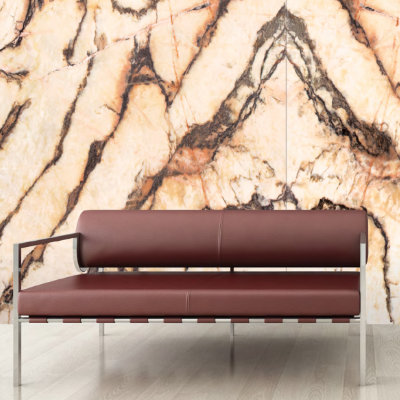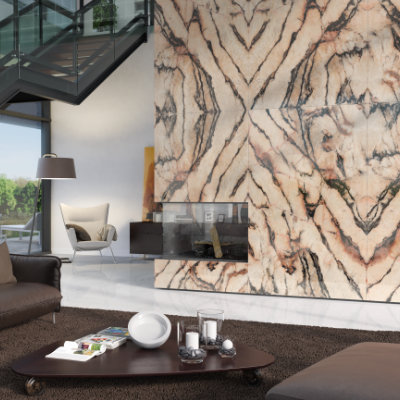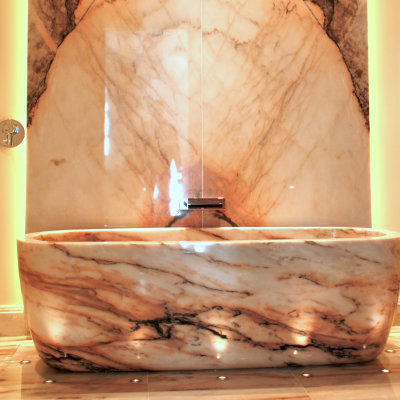# Picasso

Picasso is an extraordinary marble. Like a painting genius, the extravagant lines of its veins combine with the explosive tones of fire to create surprising and captivating ambiences. The symmetry application of Picasso's plates creates true masterpieces that leave no one indifferent. And thanks to the low opacity of the stone, the use of backlighting transforms this marble into a hypnotic and dazzling canvas.

## Examples of UsePolished | Honed

## Physical and Mechanical Characteristics

Petrographic Description
(EN 12407:2000)
Marble

Fire Behavior (reserved for uses subject to fire regulations)
(EN 13501-1:2007)
Class A1 (according to the Amendment to Decision 96/603/EC)

Flexural Strenght under Centered Load [MPa]
(EN 12372:2006)
Average value = 15.9
Standard Deviation = 0.9

Water Absorption at Atmospheric Pressure [%]
(EN 13755:2008)
Average value = 0.1
Standard Deviation = 0.0

Apparent Volume [kg.m-3]
(EN 1936:2006)
Average value = 2710
Standard Deviation = 0

Open Porosity [%]
(EN 1936:2006)
Average value = 0.2
Standard Deviation = 0.0

Abrasion Test (Capon) [mm]
(EN 14157:2004)
Average value = 21.5
Standard Deviation = 1.2

Slip Resistance [SRV]
(EN 14231:2003)
Dry = 71
Wet = 65

Resistance to Ageing by Thermal Shock (outdoor use only) [%]
(EN 14066:2003)
Mass Variation/Average value = 0.01
Mass Variation/Standard Deviation = 0.01
Decrease of Dynamic Elastic Modulus/Average value = 25.8
Decrease of Dynamic Elastic Modulus/Standard Deviation = 3.2

## Recommended Use### Interior Floor